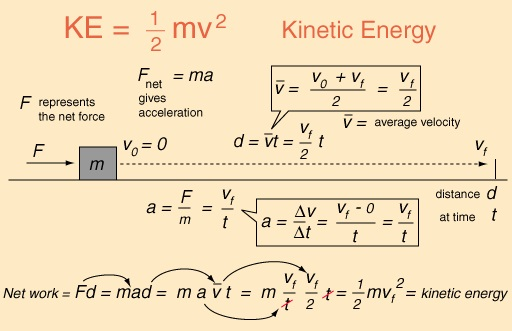# Classical Physics II – Force and Kinetic Energy

## Newton’s 1st Law of MotionNewton’s First Law of Motion states that “Every object persists in its state of rest or uniform motion in a straight line, unless it is compelled to change that state by forces impressed on it.

So, any object in motion will continue moving at a constant speed in a straight line, unless acted upon by an unbalanced force.  In the same token, any stationary object will remain at rest, unless acted upon by a force.

The energy transferred to an object by a force is an important quantity and is called the work done by the force on the object.  The greater the force that is applied, the greater the work done, and the greater the energy transferred to the object on which the force acts.

So the work$W$ done by the force is proportional to the magnitude$F$ of the force:$W \propto F$.

##Kinetic Energy: The Energy of Motion

Newton’s Second Law of Motion states that the magnitude$F$ of the force acting on an object in motion is related to its mass m and the magnitude a of its acceleration by the equation$F = ma$.

The kinetic energy$E_k$ of an object equals its mass$m$ multiplied by its acceleration$a$ multiplied by the distance$d$ travelled by the object.

This gives the equation for the kinetic energy:$E_k = mad$Kinetic Energy Explained $($Image: Hyperphysics$)$

In terms of mass$m$ and speed$v$, the kinetic energy$E_k$ of a moving object is$E_k = \frac {1}{2} mv^2$

Note. You will use this equation again and again throughout your study of Physics, so the kinetic energy is a useful and simple equation to memorize.

Now, let’s see how it goes with a worked example.  Let’s calculate the kinetic energy of a car, with mass$m = 800 kg$, and travelling at a speed of$20 ms^{-1}$.

To calculate the kinetic energy, you simply need to substitute the relevant values into the equation.

This gives$E_k = \frac{1}{2} \times 800 kg \times (20 ms^{-1})^2 = 400 kg \times 400 m^2 s^{-2} = 1.6 \times 10^5 kg m^2 s^{-2}$.

At this point, you may think: “Wrong answer!

The result is in$kg m^2 s^{-2}$.  Energy must be in Joules!”

But wait, that’s okay.  It just so happens that$1 Joule = 1 kg m^2 s^{-2}$.

So, we’re good after all.  Phew!

The kinetic energy of the moving car is equal to$1.6 \times 10^5 J$.

## Little 'Bytes' about Natural Phenomena, Theoretical Physics and the Latest Worldwide Scientific Findings. Edited from Glasgow, Scotland.